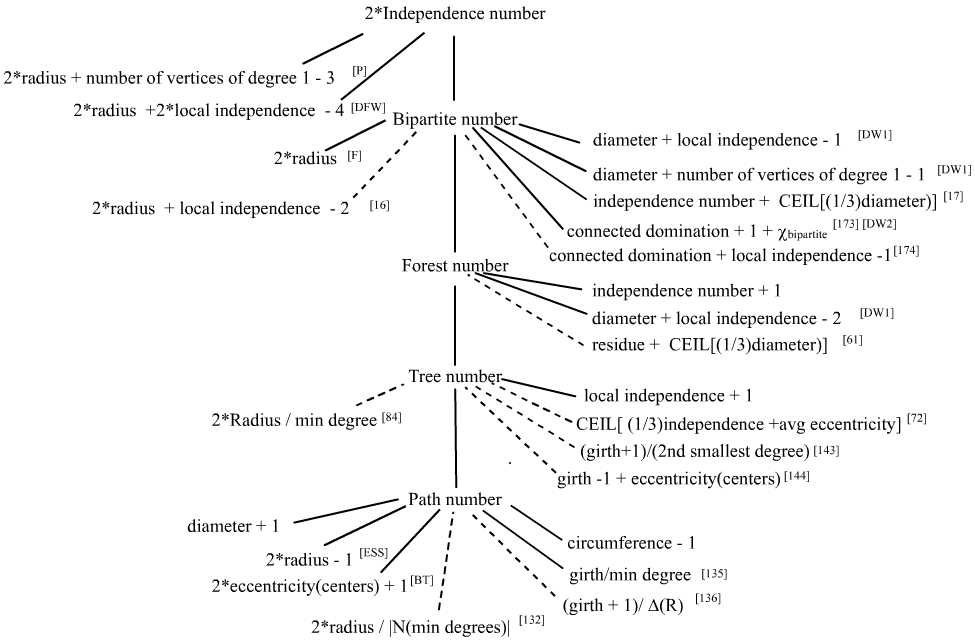Written on the Wall II (Conjectures of Graffiti.pc)

Ermelinda DeLaVina (delavinae@uhd.edu)

About the program On Some Conjectures
Written on the Wall II is composed mainly of conjectures of my conjecture-making program Graffiti.pc (G.pc for short) which was inspired by Siemion Fajtlowicz's conjecture-making program Graffiti. Both programs utilize Fajtlowicz's Dalmatian heuristic, however each has its individual implementations. The first 8 conjectures were generated by Graffiti, while I was Fajtlowicz's student, see 1996 list(pdf) for the original list. The remaining conjectures on this list (as of 2001) were generated by Graffiti.pc. Please send information to delavinae@uhd.edu on any of the conjectures on this list.

Related websites and papers

Siemion Fajtlowicz's list (with comments) Written on the Wall is available from him by request. Also available is bibliographical information on papers inspired by conjectures of Graffiti, since it inception in the mid-1980s; that webpage includes a variety of papers related to Graffiti.

Some of the most recent papers that describe and discuss Graffiti include:

 E. DeLaVina, On Some History of the Development of Graffiti (ps) (pdf), Graphs and Discovery DIMACS: Series in Discrete Mathematics and Theoretical Computer Science, 69(2005), 81-118.S. Fajtlowicz, Toward Fully Automated Fragments of Graph Theory II, (pdf). C. E. Larson, A Survey in Automated Mathematical Conjecture-Making (pdf), Graphs and Discovery DIMACS: Series in Discrete Mathematics and Theoretical Computer Science, 69(2005), 297-318..

Papers describing Graffiti.pc include:

 section 5 of the above paper "On Some History of the Development of Graffiti" and E. DeLaVina, Graffiti.pc: A Variant of Graffiti, (pdf), Graphs and Discovery DIMACS: Series in Discrete Mathematics and Theoretical Computer Science, 69(2005), 71-79.
At the moment, a majority of conjectures on Written on Wall II are lower bounds on the maximum order of certain induced subgraphs of a connected graph.  The bipartite number of a graph is the order of a largest induced bipartite subgraph of the graph; the forest, tree and path numbers are the orders of largest induced forests, trees and paths, resp., of a graphs.

The diagram below (you may have to scroll down) illustrates the relationship between (by means of a solid line) the bipartite, forest, tree and path numbers, namely that bipartite number ≥ forest number ≥ tree number ≥ path number. The diagram presents other correct relations also through solid lines using the convention that a line emanating from an invariant down to an expression of invariants represents the relation greater than or equal to. For instance, it is known that for a simple connected graph, the path number ≥ 2*radius - 1 (a result of F. Chung in [ESS]). On the other hand, a dashed line indicate a conjectured relation between the expressions. For instance, G.pc's conjecture #17 is that  for a simple connected graph, the bipartite number ≥ independence number + CEIL[(1/3)diameter] (to see all open conjectures of G.pc click on the menu on the left). Relations that are not referenced with a paper or conjecture number are simple exercises, such as bipartite number ≥ forest number ≥ tree number ≥ path number ≥ diameter + 1; such relations are listed in order to emphasize that many of G.pc's conjectures on maximum induced subgraphs propose improvements on these simple propositions.

References

 [BT] G. Bacso and Z. Tuza, A characterization of graphs without long induced paths, Journal of Graph Theory, 14(1990), 455-464. [DFW] E. DeLaVina, S. Fajtlowicz and B. Waller, On Some conjectures of Griggs and Graffiti, Graphs and Discovery DIMACS: Series in Discrete Mathematics and Theoretical Computer Science, 69(2005), 119-125. [DW1] E. DeLaVina and B. Waller, On some conjectures of graffiti.pc on the maximum order of induced subgraphs (pdf), Congressus Numerantium, 2004 [DW2] E. DeLaVina and B. Waller, Spanning trees with many leaves and the maximum order of bipartite Subgraphs, 2005 (preprint) [ESS] P. Erdos,, M. Saks, and V. Sos, Maximum induced trees in graphs, Journal of Graph Theory, 41(1986), p. 61-79. [F] S. Fajtlowicz, A characterization of radius-critical graphs, Journal of Graph Theory, 12(1988), p. 529-532. [P] R. Pepper, ...., Preprintmy homepage